Author: Oscar Cronquist Article last updated on September 05, 2022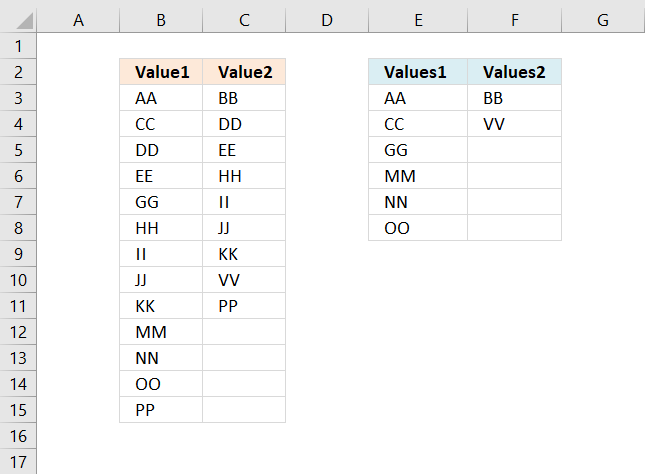This article demonstrates formulas that extract values that exist only in one column out of two columns.

There are text values in column B and column C.

## 1. Compare two columns and extract differences - Excel 365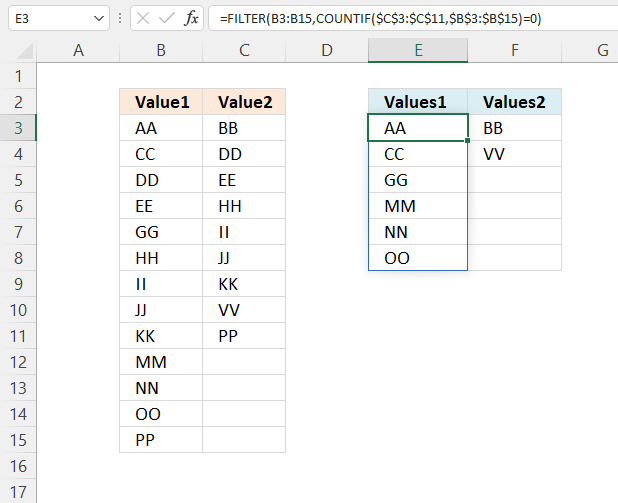The formula in cell E3 extracts values in cell range B3:B15 that are not in cell range C3:C11, meaning they exist only in cell range B3:B15.

For example, value "AA" in cell B3 is not in cell range C3:C11, however, value "DD" in cell B5 is also in cell range C3:C11, in cell C4.

Excel 365 formula in cell E3:

=FILTER(B3:B15,COUNTIF(C3:C11,B3:B15)=0)

The formula in cell F3 extracts values in cell range C3:C11 that are not in cell range B3:B15

Excel 365 formula in cell F3:

=FILTER(C3:C11,COUNTIF(B3:B15,C3:C11)=0)

The dynamic Excel 365 formulas above are entered like regular formulas.

## Explaining formula

#### Step 1 - Compare/Count values between C3:C11 and B3:B15

The COUNTIF function calculates the number of cells that is equal to a condition.

Function syntax: COUNTIF(range, criteria)

COUNTIF(C3:C11, B3:B15)

becomes

COUNTIF({"BB"; "DD"; "EE"; "HH"; "II"; "JJ"; "KK"; "VV"; "PP"},{"AA"; "CC"; "DD"; "EE"; "GG"; "HH"; "II"; "JJ"; "KK"; "MM"; "NN"; "OO"; "PP"})

and returns

{0; 0; 1; 1; 0; 1; 1; 1; 1; 0; 0; 0; 1}.

#### Step 2 - Check if a number is equal to zero

The equal sign lets you compare value to value, it is also possible to compare multiple values to a value. The equal sign is a logical operator and returns a boolean value TRUE or FALSE.

COUNTIF(C3:C11,B3:B15)=0

becomes

{0; 0; 1; 1; 0; 1; 1; 1; 1; 0; 0; 0; 1}=0

and returns

{TRUE; TRUE; FALSE; FALSE; TRUE; FALSE; FALSE; FALSE; FALSE; TRUE; TRUE; TRUE; FALSE}.

#### Step 3 - Filter values based on boolean values

The FILTER function extracts values/rows based on a condition or criteria.

Function syntax: FILTER(array, include, [if_empty])

FILTER(B3:B15, COUNTIF(C3:C11, B3:B15)=0)

becomes

FILTER(B3:B15, {TRUE; TRUE; FALSE; FALSE; TRUE; FALSE; FALSE; FALSE; FALSE; TRUE; TRUE; TRUE; FALSE})

becomes

FILTER({"BB"; "DD"; "EE"; "HH"; "II"; "JJ"; "KK"; "VV"; "PP"},{"AA"; "CC"; "DD"; "EE"; "GG"; "HH"; "II"; "JJ"; "KK"; "MM"; "NN"; "OO"; "PP"}, {TRUE; TRUE; FALSE; FALSE; TRUE; FALSE; FALSE; FALSE; FALSE; TRUE; TRUE; TRUE; FALSE})

and returns

{"AA";"CC";"GG";"MM";"NN";"OO"}.

## 2. Compare two columns and extract differences - earlier versions##### Array formula for older Excel versions

The array formula in cell E3 extracts values existing only in column B, compared to column C:

=INDEX(\$B\$3:\$B\$15, SMALL(IF(COUNTIF(\$C\$3:\$C\$11, \$B\$3:\$B\$15)=0, MATCH(ROW(\$B\$3:\$B\$15), ROW(\$B\$3:\$B\$15)), ""), ROWS(\$A\$1:A1)))

The array formula in cell F3 extracts values existing only in column C, compared to column B:

=INDEX(\$C\$3:\$C\$11,SMALL(IF(COUNTIF(\$B\$3:\$B\$15,\$C\$3:\$C\$11)=0,MATCH(ROW(\$C\$3:\$C\$11),ROW(\$C\$3:\$C\$11)),""),ROWS(\$A\$1:A1)))

#### How to enter array formula in cell E3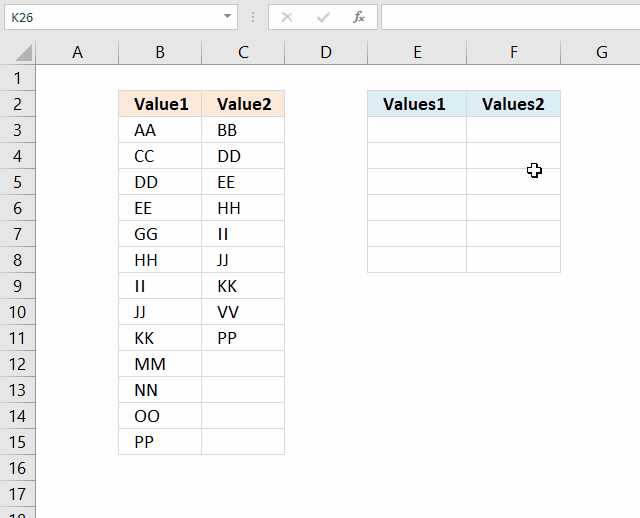1. Copy above array formula (Ctrl + c).
2. Select cell E3.
3. Press with left mouse button on in the formula bar.
4. Paste array formula (Ctrl + v) to the formula bar.
5. Press and hold CTRL + SHIFT simultaneously.
6. Press Enter once.
7. Release all keys.

The formula is now an array formula. See the curly brackets, they tell you it is an array formula. Don't enter the curly brackets yourself, they appear if you enter it correctly, like this:

{=INDEX(\$A\$2:\$A\$14, SMALL(IF(COUNTIF(\$B\$2:\$B\$10, \$A\$2:\$A\$14)=0, MATCH(ROW(\$A\$2:\$A\$14), ROW(\$A\$2:\$A\$14)), ""), ROW(A1)))}

#### How to copy array formula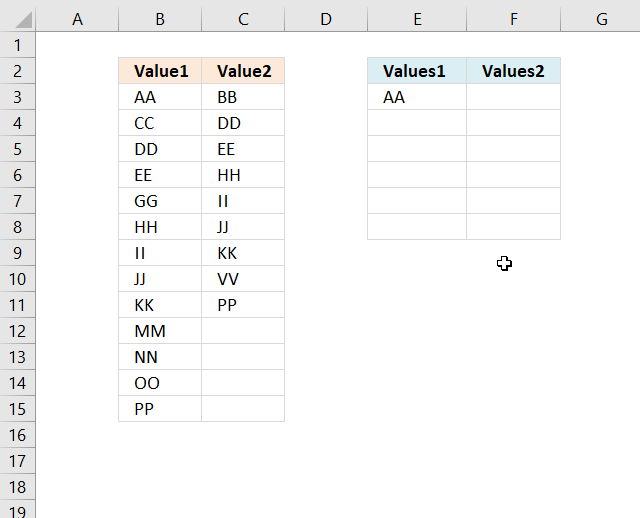1. Select cell E3.
2. Copy (Ctrl + c).
3. Select cell range E4:E8.
4. Paste (Ctrl + v).

### Explaining array formula in cell E3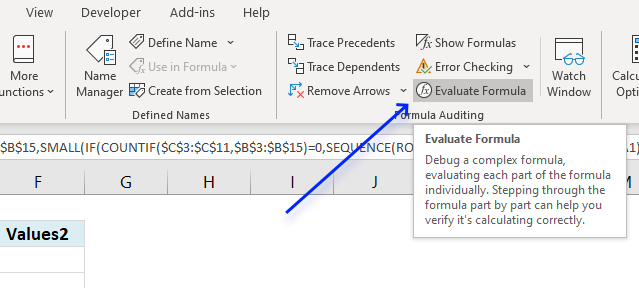I recommend the "Evaluate Formula" tool when you want to understand, troubleshoot or examine a specific formula.

Select the cell containing the formula you want to evaluate. Go to tab "Formulas" on the ribbon, press with left mouse button on the "Evaluate Formula" button, see image above.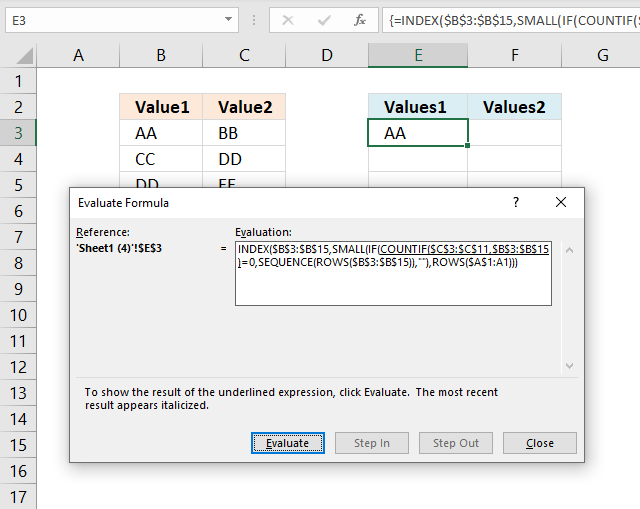A dialog box appears, it shows the formula and the button "Evaluate" below the formula allows you to go through the formula calculations step by step.

##### Step 1 - Count values in column C based on values in column B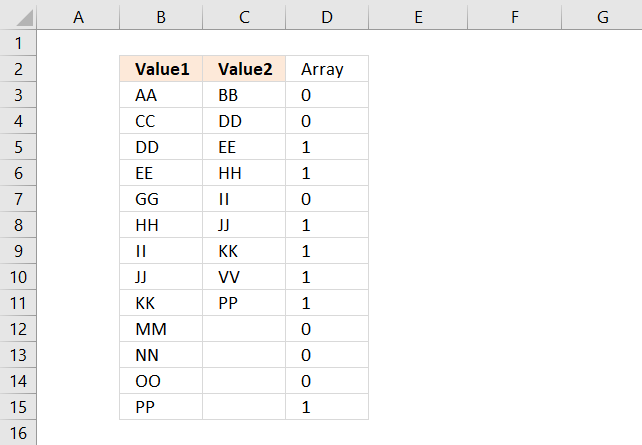The COUNTIF function lets you count values based on a condition, however, it is also possible to use multiple conditions but then the function returns an array of values instead of a single value.

This is what makes the formula an array formula. Here are the arguments in the COUNTIF function:

COUNTIF(rangecriteria)

COUNTIF(\$C\$3:\$C\$11, \$B\$3:\$B\$15)

becomes

COUNTIF({"BB"; "DD"; "EE"; "HH"; "II"; "JJ"; "KK"; "VV"; "PP"}, \$B\$3:\$B\$15)

becomes

COUNTIF({"BB"; "DD"; "EE"; "HH"; "II"; "JJ"; "KK"; "VV"; "PP"}, {"AA"; "CC"; "DD"; "EE"; "GG"; "HH"; "II"; "JJ"; "KK"; "MM"; "NN"; "OO"; "PP"})

and returns the following array of values:

{0; 0; 1; 1; 0; 1; 1; 1; 1; 0; 0; 0; 1}

The position of each value in the array is very important, they make it possible to identify and extract the values we want. The position of each value in the array corresponds to the value in column B, see image above.

A 0 (zero) means that the value in column B is not found in column C. 1 is that the value in column B is found once in column C.

##### Step 2 - Check if they are equal to 0 (zero)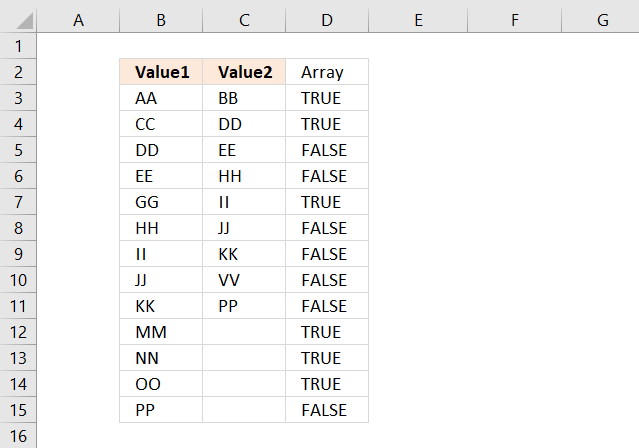The equal sign checks if the values are equal to 0 (zero) and returns the boolean values TRUE or FALSE.

COUNTIF(\$C\$3:\$C\$11, \$B\$3:\$B\$15)=0

becomes

{0; 0; 1; 1; 0; 1; 1; 1; 1; 0; 0; 0; 1}=0

and returns

{TRUE; TRUE; FALSE; FALSE; TRUE; FALSE; FALSE; FALSE; FALSE; TRUE; TRUE; TRUE; FALSE}

##### Step 3 - If they are equal to zero, return the corresponding relative row number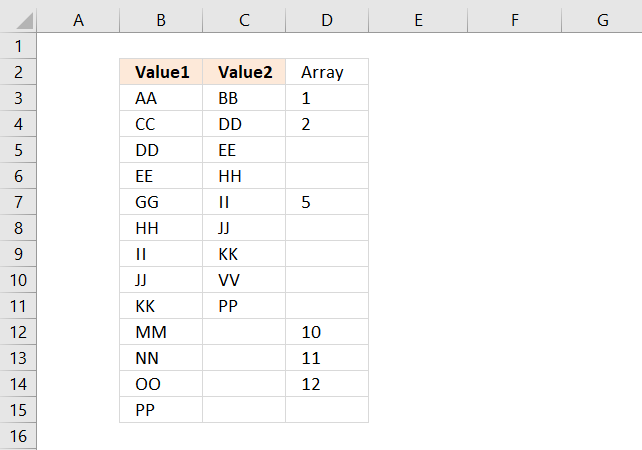The IF function allows you to return a specific value if the logical test is TRUE and another value if FALSE.

IF(logical_test, [value_if_true], [value_if_false])

IF(COUNTIF(\$C\$3:\$C\$11, \$B\$3:\$B\$15)=0, MATCH(ROW(\$B\$3:\$B\$15), ROW(\$B\$3:\$B\$15)), "")

becomes

IF({TRUE; TRUE; FALSE; FALSE; TRUE; FALSE; FALSE; FALSE; FALSE; TRUE; TRUE; TRUE; FALSE}, MATCH(ROW(\$B\$3:\$B\$15), ROW(\$B\$3:\$B\$15)), "")

The MATCH and ROW functions create an array from 1 to 11 which we then will use to extract the correct value from cell range B3:B15.

MATCH(ROW(\$B\$3:\$B\$15), ROW(\$B\$3:\$B\$15))

becomes

MATCH({3; 4; 5; 6; 7; 8; 9; 10; 11; 12; 13; 14; 15}, {3; 4; 5; 6; 7; 8; 9; 10; 11; 12; 13; 14; 15})

and returns

{1; 2; 3; 4; 5; 6; 7; 8; 9; 10; 11; 12; 13}.

##### Step 4 - Return the k-th smallest row number

The SMALL function returns the k-th smallest number from an array or cell range.

SMALL(IF(COUNTIF(\$C\$3:\$C\$11, \$B\$3:\$B\$15)=0, MATCH(ROW(\$B\$3:\$B\$15), ROW(\$B\$3:\$B\$15)), ""), ROWS(\$A\$1:A1))

beomes

SMALL({1; 2; 3; 4; 5; 6; 7; 8; 9; 10; 11; 12; 13}, ROWS(\$A\$1:A1))

The ROWS function counts the number of rows in a given cell reference. The cell ref in this example expands when you copy the cell and paste to cells below. This makes the SMALL function return a new number in each cell.

SMALL({1; 2; 3; 4; 5; 6; 7; 8; 9; 10; 11; 12; 13}, ROWS(\$A\$1:A1))

becomes

SMALL({1; 2; 3; 4; 5; 6; 7; 8; 9; 10; 11; 12; 13}, 1)

and returns 1.

##### Step 5 - Return value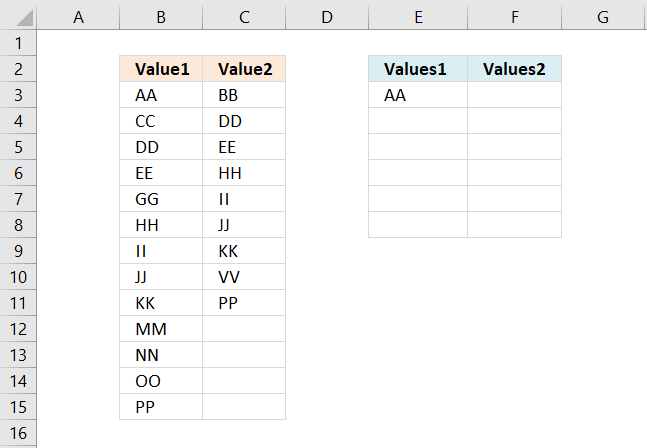The INDEX function returns a value or multiple values based on a row and/or column number.

INDEX(\$B\$3:\$B\$15, SMALL(IF(COUNTIF(\$C\$3:\$C\$11, \$B\$3:\$B\$15)=0, MATCH(ROW(\$B\$3:\$B\$15), ROW(\$B\$3:\$B\$15)), ""), ROWS(\$A\$1:A1)))

becomes

INDEX(\$B\$3:\$B\$15, 1)

and returns "AA" in cell E3.